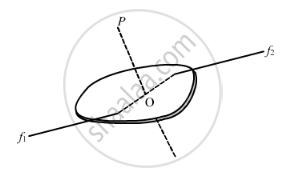Advertisement Remove all ads

# Particles of Masses 1 G, 2 G, 3 G, .........., 100 G Are Kept at the Marks 1 Cm, 2 Cm, 3 Cm, ..........., 100 Cm Respectively on a Metre Scale. - Physics

Sum

Particles of masses 1 g, 2 g, 3 g, .........., 100 g are kept at the marks 1 cm, 2 cm, 3 cm, ..........., 100 cm respectively on a metre scale. Find the moment of inertia of the system of particles about a perpendicular bisector of the metre scale.

Advertisement Remove all ads

#### Solution

It is given that the perpendicular bisector of the metre scale is passed through the 50thparticle.

Therefore, on the L.H.S. of the axis, there will be 49 particles and on the R.H.S., there will be 50 particles.

Consider the two particles positioned at 49 cm and 51 cm.

Moment of inertia due to these two particles = 49 × (1)2 + 51 × (1)2

I1 = 100 × 1 = 100 gm-cm2

Similarly, if we consider particles positioned at 48 cm and 52 cm, we get

I2  = 100 × (2)2 gm-cm2

Thus, we will get 49 such sets and one particle at 100 cm. Therefore, total moment of inertia,

$I = \left( I_1 + I_2 + I_3 . . . . . + I_{49} \right) + I'$

Here, I' is the moment of inertia of particle at 100 cm.

$So, I = 100 \left( 1^2 + 2^2 + 3^2 + . . . + {49}^2 \right) + 100 \left( 50 \right)^2$

$= 100 \left( 1^2 + 2^2 + 3^2 + . . . + {50}^2 \right)$

$= 100 \times \frac{\left( 50 \times 51 \times 101 \right)}{6}$

$= 100 \times 25 \times 17 \times 101 = 4292599 gm - {cm}^2$

Or, I = 0.429 kg-m2 ≃ 0.43 kg-m2Concept: Values of Moments of Inertia for Simple Geometrical Objects (No Derivation)
Is there an error in this question or solution?
Advertisement Remove all ads

#### APPEARS IN

HC Verma Class 11, 12 Concepts of Physics 1
Chapter 10 Rotational Mechanics
Q 10 | Page 196
Advertisement Remove all ads
Advertisement Remove all ads
Share
Notifications

View all notifications

Forgot password?